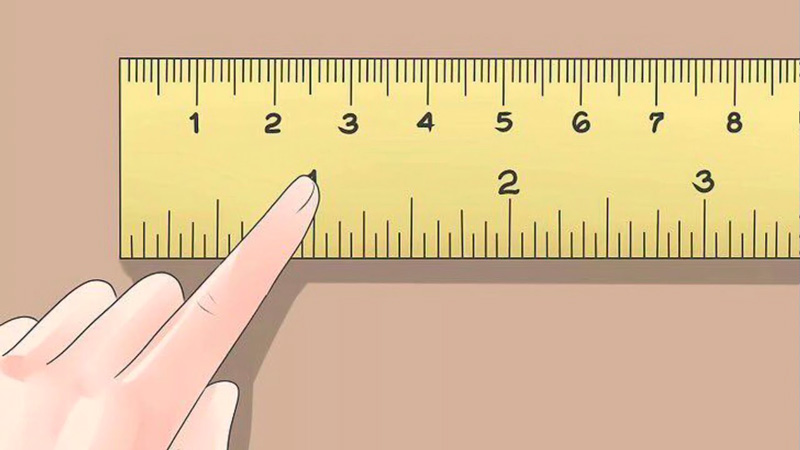One inch equals two whole and fifty-four hundredths of a centimeter. The most famous legend of the origin of this unit of measurement is that an inch is the approximate width of the thumb. It is designated as two top dashes (“). A centimeter is a metric measure equal to one hundredth of a meter. Here you can convert centimeters to inches using our simple and handy online calculator. It is free and fast! Such a calculator will be very useful if you live in a country that uses the imperial or British system of measures.Visual comparison of a centimeter and an inch

CalcuLife.com